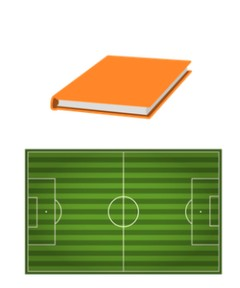Understanding what area is

# Understanding what area is

Students learn to understand what area is.

No account needed.8,000 schools use Gynzy92,000 teachers use Gynzy1,600,000 students use Gynzy

## General

The students learn what area is and when to use it.

## Standards

CCSS.Math.Content.3.MD.C.5

## Learning objective

Students will be able to tell what the concept of area means (understanding).

## Introduction

On the interactive whiteboard there are pairs of images. The students must determine which is larger in real life. For example, a soccer field is larger than a book.

## Instruction

You first explain that area tells how large a surface is. Then the students can experience what area is for themselves by covering their own table/desk with books or notebooks. You explain that the part of the table that they just covered is the area of the table. Make sure that students don't confuse area with perimeter. Next you have students look at various shapes and determine what color the area is. After this you explain that when you use area, you want to know how large an area, or surface, is. For example if you want to know what the area is of a wall that you are going to paint. For the following statements, the students must determine whether each statement is true or false.

To check whether the students know what area is and then to use it, you can ask the following questions:
- What color is the area of this shape?
- Can you name an example of the area of something?
- What has the larger area: a soccer field or a classroom?

## Quiz

The students test their understanding of what area is through ten exercises. In some of the exercises the students must identify which color the area is on a shape, and the other exercises are statements about what area is or how it is used. The students must determine whether these statements are true or false.

## Closing

You recall the learning goal and its importance. Next there are two children that are each making a statement about the area of the shape. The students must determine which of the children is correct. This is repeated with two children that are making a statement about what area is used for.

## Teaching tips

When students have difficulty with this learning goal, you can emphasize that the word area means the size of the area, or the surface that you are measuring.

## Instruction materials

Notebooks or books

### The online teaching platform for interactive whiteboards and displays in schools

• Save time building lessons

• Manage the classroom more efficiently

• Increase student engagement